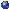Let X be a topological space, and let n be a positive integer. The n-stranded configuration space of X is the topological space F_n(X) that parametrizes unordered n-tuples of distinct points in X. One of the earliest results in homological stability states that if X is an orientable manifold with boundary, then the isomorphism type of the cohomology groups H^i(F_n(X)) only depends on i whenever n is sufficiently large with respect to i. One of the earliest questions that the speaker was asked by his advisor was whether this result could be expanded to cases wherein X is a graph, i.e. a one-dimensional CW-complex. While the answer to this question turns out to be "no," what is actually true is perhaps more interesting, requiring tools from graph theory, commutative algebra, and even category theory to prove. In this talk I will lightly introduce the aforementioned classical theorems in homological stability, before moving on to the kinds of behaviors exhibited by graph configuration spaces as you increase the number of strands. Next, I will discuss the newly discovered answer to the perpendicular question of "what behaviors do these spaces exhibit when you fix the number of strands, but allow the graph itself to vary in some nice way," as well as how these two orthogonal perspectives can be made to fit nicely into one single theory. This talk spans about five years of work, covering papers of the speaker, as well as Miyata, Proudfoot, and the speaker.